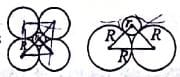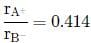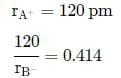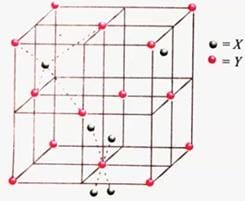NEET  >  Test: Number of Atoms in a Unit Cell & Closed-Packed Structures (NCERT)

# Test: Number of Atoms in a Unit Cell & Closed-Packed Structures (NCERT) - NEET

Test Description

## 25 Questions MCQ Test Chemistry Class 12 - Test: Number of Atoms in a Unit Cell & Closed-Packed Structures (NCERT)

Test: Number of Atoms in a Unit Cell & Closed-Packed Structures (NCERT) for NEET 2023 is part of Chemistry Class 12 preparation. The Test: Number of Atoms in a Unit Cell & Closed-Packed Structures (NCERT) questions and answers have been prepared according to the NEET exam syllabus.The Test: Number of Atoms in a Unit Cell & Closed-Packed Structures (NCERT) MCQs are made for NEET 2023 Exam. Find important definitions, questions, notes, meanings, examples, exercises, MCQs and online tests for Test: Number of Atoms in a Unit Cell & Closed-Packed Structures (NCERT) below.
Solutions of Test: Number of Atoms in a Unit Cell & Closed-Packed Structures (NCERT) questions in English are available as part of our Chemistry Class 12 for NEET & Test: Number of Atoms in a Unit Cell & Closed-Packed Structures (NCERT) solutions in Hindi for Chemistry Class 12 course. Download more important topics, notes, lectures and mock test series for NEET Exam by signing up for free. Attempt Test: Number of Atoms in a Unit Cell & Closed-Packed Structures (NCERT) | 25 questions in 25 minutes | Mock test for NEET preparation | Free important questions MCQ to study Chemistry Class 12 for NEET Exam | Download free PDF with solutions
 1 Crore+ students have signed up on EduRev. Have you?
Test: Number of Atoms in a Unit Cell & Closed-Packed Structures (NCERT) - Question 1

### For the structure given below the site marked as S is aDetailed Solution for Test: Number of Atoms in a Unit Cell & Closed-Packed Structures (NCERT) - Question 1

Octahedral voids occupy the position of edge centre and body centre.

Test: Number of Atoms in a Unit Cell & Closed-Packed Structures (NCERT) - Question 2

### Which of the following statements is not about the voids?

Detailed Solution for Test: Number of Atoms in a Unit Cell & Closed-Packed Structures (NCERT) - Question 2

Octahedral sites are created by overlapping two equilateral triangles with apices in opposite directions.

Test: Number of Atoms in a Unit Cell & Closed-Packed Structures (NCERT) - Question 3

### In ABC packing if the number of atoms in the unit cell is n then the number of tetrahedral voids in the unit cell is equal to:

Detailed Solution for Test: Number of Atoms in a Unit Cell & Closed-Packed Structures (NCERT) - Question 3

Number of tetrhedral voids per atom in a unit cell is 2.
Hence the total numberof tetrahedral voids =2n.

Test: Number of Atoms in a Unit Cell & Closed-Packed Structures (NCERT) - Question 4

If the radius of an octahedral void is r and the radius of atoms in close packing is R, then the relation between r and R is :

Detailed Solution for Test: Number of Atoms in a Unit Cell & Closed-Packed Structures (NCERT) - Question 4(2R)2 = (R + r)2 + (R + r)2
√2 R = R + r
(√2 - 1)R = r
0.414R = r

Test: Number of Atoms in a Unit Cell & Closed-Packed Structures (NCERT) - Question 5

A metal crystallises into a lattice containing a sequence of layers as AB AB AB ... . What percentage of voids are left in the lattice?

Detailed Solution for Test: Number of Atoms in a Unit Cell & Closed-Packed Structures (NCERT) - Question 5

ABAB packing is hexagonal closepacking in which all atoms occupy 74% of the total space. Hence, 26% of voids are left in the lattice.

Test: Number of Atoms in a Unit Cell & Closed-Packed Structures (NCERT) - Question 6

In ccp arrangement the pattern of successive layers can be designated as

Detailed Solution for Test: Number of Atoms in a Unit Cell & Closed-Packed Structures (NCERT) - Question 6

In ccp pattern, fourth layer is identical to first layer.

Test: Number of Atoms in a Unit Cell & Closed-Packed Structures (NCERT) - Question 7

Which of the following statements is not correct about hexagonal close packing?

Detailed Solution for Test: Number of Atoms in a Unit Cell & Closed-Packed Structures (NCERT) - Question 7

The coordination number is 12

Test: Number of Atoms in a Unit Cell & Closed-Packed Structures (NCERT) - Question 8

A crystalline structure has radius ratio (r+/r-)  in the range of 0.225 - 0.414. The coordination number and arrangement of anions around the cations are:

Detailed Solution for Test: Number of Atoms in a Unit Cell & Closed-Packed Structures (NCERT) - Question 8

If the radius ratio is between 0.225 and 0.414, it will have a tetrahedral void. The derivation of this actually takes place in reverse where we assume a tetrahedral void and arrive at the value, but the converse also holds true. A tetrahedral void naturally means there are 4 surrounding atoms. So, coordination number will be 4.

Test: Number of Atoms in a Unit Cell & Closed-Packed Structures (NCERT) - Question 9

A Crystal lattice with alternate +ve "and "-veions has radius ratio of 0.524. The coordination number of lattice is

Detailed Solution for Test: Number of Atoms in a Unit Cell & Closed-Packed Structures (NCERT) - Question 9

Coordination number of crystal lattices which fall in the range of 0.414 - 0.732 is 6.

Test: Number of Atoms in a Unit Cell & Closed-Packed Structures (NCERT) - Question 10

A solid AB has a rock salt structure. If radius of cation A+ is 120 pm, what is the minimum value of radius of B anion?

Detailed Solution for Test: Number of Atoms in a Unit Cell & Closed-Packed Structures (NCERT) - Question 10

Limited Ratio⟹rB− = 290 pm

Test: Number of Atoms in a Unit Cell & Closed-Packed Structures (NCERT) - Question 11

A crystal formed by two elements X and Y in cubic structure. X atoms are at the corners of cube while Y atoms are at the face center. The formula of he compound will be:

Detailed Solution for Test: Number of Atoms in a Unit Cell & Closed-Packed Structures (NCERT) - Question 11

No. of X atom (at the corners) = 1/8 x 8 = 1
No. of Y atoms (fcc) = 6 × 1/2 = 3
hence, the formula is XY

Test: Number of Atoms in a Unit Cell & Closed-Packed Structures (NCERT) - Question 12

If three elements X,Y and Z crystallizes in a ccp lattice with the X atoms at the corner, Y atoms at the cube centre and Z atom at the edge centre the formula of the compound will be:

Detailed Solution for Test: Number of Atoms in a Unit Cell & Closed-Packed Structures (NCERT) - Question 12

No. of X atoms = 1/8 x 8 = 1
No. of Y atoms = 1
No. of Z atoms = 12 x 1/4 = 3
Formula of the compound = XYZ3

Test: Number of Atoms in a Unit Cell & Closed-Packed Structures (NCERT) - Question 13

A compound is formed by two elements Y and Z. The element Z forms ccp and atoms Y occupy 1/3rd of tetrahedral voids. The formula of the compound is

Detailed Solution for Test: Number of Atoms in a Unit Cell & Closed-Packed Structures (NCERT) - Question 13

Suppose no. of atoms of Z = a

No. of tetrahedral voids = 2a

No. of atoms of Y = 1/3 × 2a = 2a/3

Ratio of atoms of y and Z= 2a/3 : a = 2:3

Hence, the formula of the compound = Y2Z3

Test: Number of Atoms in a Unit Cell & Closed-Packed Structures (NCERT) - Question 14

A cubic solid is made up of two elements P and Q. Atoms of P are present at the corners of the cube and atoms of Q are present by body centre. What is the formula of the compound and what are coordination number of P and Q?

Detailed Solution for Test: Number of Atoms in a Unit Cell & Closed-Packed Structures (NCERT) - Question 14

No. of atoms of P = 8 x 1/8 = 1
No. of atoms of Q = 1 x 1 = 1
The formula of the compound is PQ. Coordination no. of P and Q = 8:8

Test: Number of Atoms in a Unit Cell & Closed-Packed Structures (NCERT) - Question 15

How many chloride ions are surrounding sodium ion in a sodium chloride crystal?

Detailed Solution for Test: Number of Atoms in a Unit Cell & Closed-Packed Structures (NCERT) - Question 15

Each Na+ ion is surrounded by 6 Cl-ions and each Cl- ion is surrounded by 6 Na+ ions in NaCl crystal.

Test: Number of Atoms in a Unit Cell & Closed-Packed Structures (NCERT) - Question 16

In NaCl structure,

Detailed Solution for Test: Number of Atoms in a Unit Cell & Closed-Packed Structures (NCERT) - Question 16

Octahedral sites are occupied by Na+ ions in NaCl structure.

Test: Number of Atoms in a Unit Cell & Closed-Packed Structures (NCERT) - Question 17

NaCl type crystal (with coordination no. 6:6) can be converted into CsCl type crystal (with coordination no. 8:8) by applying:

Detailed Solution for Test: Number of Atoms in a Unit Cell & Closed-Packed Structures (NCERT) - Question 17

Increase of pressure increases coordination number. Hence, by applying high pressure, NaCl type crystal can be changed into CsCl type crystal

Test: Number of Atoms in a Unit Cell & Closed-Packed Structures (NCERT) - Question 18

Coordination number of Cs+ and Cl- in CsCl crystal are:

Detailed Solution for Test: Number of Atoms in a Unit Cell & Closed-Packed Structures (NCERT) - Question 18

CsCl has a bcc structure. Each Cs ion is surrounded by 8 Cl ions and each Cl ion is surrounded by 8 Cs+ ions. CsCl has a bcc type structure. Thus, the structure has 8 : 8 coordination number.

Test: Number of Atoms in a Unit Cell & Closed-Packed Structures (NCERT) - Question 19

In zinc blende structure,

Detailed Solution for Test: Number of Atoms in a Unit Cell & Closed-Packed Structures (NCERT) - Question 19

ZnS has a ccp structure. S2− ions are present at the corners of the cube and centre of each face. Zn2+ ions occupy half of the tetrahedral sites. Each Zn2+ ion is surrounded by four S2− ions and each S2− ion is surrounded by four Zn2+ ions.

Test: Number of Atoms in a Unit Cell & Closed-Packed Structures (NCERT) - Question 20

Consider the unit cell given below :The unit cell shown in the figure belongs to

Detailed Solution for Test: Number of Atoms in a Unit Cell & Closed-Packed Structures (NCERT) - Question 20

ZnS has a ccp structure. S2- ions at the corners of the cube and centre of each face. Zn2+ ions occupy half of the tetrahedral sites. Each Zn2+ ion is surrounded by four S2- ions and each S2- ion is  surrounded by four Zn2+ ions.

Test: Number of Atoms in a Unit Cell & Closed-Packed Structures (NCERT) - Question 21

Consider the unit cell given below :The coordination number of Y will be

Detailed Solution for Test: Number of Atoms in a Unit Cell & Closed-Packed Structures (NCERT) - Question 21

Coordination no. of Y (Zn2+) is 4.

Test: Number of Atoms in a Unit Cell & Closed-Packed Structures (NCERT) - Question 22

Consider the unit cell given below :Which of the following about the above structure is not correct?

Detailed Solution for Test: Number of Atoms in a Unit Cell & Closed-Packed Structures (NCERT) - Question 22

Each X ion is surrounded by 4Y ions.

Test: Number of Atoms in a Unit Cell & Closed-Packed Structures (NCERT) - Question 23

In  CaF2 type (fluorite structure),  Ca2+ ions form__(A)___ structure and F ions are present in all  __(B)___ voids. The coordination number of Ca2+ is ___(C)___ and F  is___(D)____.
(A), (B), (C) and (D) respectively are:

Detailed Solution for Test: Number of Atoms in a Unit Cell & Closed-Packed Structures (NCERT) - Question 23

Ca2+ ions form ccp structure while F- ions in all tetrahedral holes and coordination numbers are 8 and 4.

Test: Number of Atoms in a Unit Cell & Closed-Packed Structures (NCERT) - Question 24

A unit of BaCl2 (fluorite structure) is made up of:

Detailed Solution for Test: Number of Atoms in a Unit Cell & Closed-Packed Structures (NCERT) - Question 24

In fluorite structure, cations form ccp structure and anions are present in all tetrahedral holes. Hence, no. of Ba2+ ions =8 and no. of Cl− ions = 4

Test: Number of Atoms in a Unit Cell & Closed-Packed Structures (NCERT) - Question 25

Which of the following structures is not correctly matched ?

Detailed Solution for Test: Number of Atoms in a Unit Cell & Closed-Packed Structures (NCERT) - Question 25

In NaCl type, Clions form ccp structures while Na+ ions are present in all octahedral voids.

## Chemistry Class 12

154 videos|396 docs|261 tests
Information about Test: Number of Atoms in a Unit Cell & Closed-Packed Structures (NCERT) Page
In this test you can find the Exam questions for Test: Number of Atoms in a Unit Cell & Closed-Packed Structures (NCERT) solved & explained in the simplest way possible. Besides giving Questions and answers for Test: Number of Atoms in a Unit Cell & Closed-Packed Structures (NCERT), EduRev gives you an ample number of Online tests for practice

## Chemistry Class 12

154 videos|396 docs|261 tests

### How to Prepare for NEET

Read our guide to prepare for NEET which is created by Toppers & the best Teachers(Scan QR code)